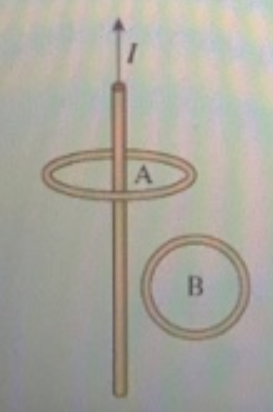# Problem: The current I in the wire in the figure shown is increasing.(a) What is the direction of the induced current in loop A?A. clockwiseB. counterclockwiseC. No current is induced in this loop(b) What is the direction of the induced current in loop B?A. clockwiseB. counterclockwiseC. No current is induced in this loop

###### FREE Expert Solution

(a) Current in the wire is increasing in the upward direction.

The current in the straight wire produces a magnetic field that is perpendicular to the wire everywhere, which means it is parallel to the plane of loop A.

Therefore, the flux through the loop A is zero.

89% (440 ratings)###### Problem Details

The current I in the wire in the figure shown is increasing.

(a) What is the direction of the induced current in loop A?

A. clockwise
B. counterclockwise
C. No current is induced in this loop

(b) What is the direction of the induced current in loop B?

A. clockwise
B. counterclockwise
C. No current is induced in this loop